# Ammeter Working Principle and Types of Ammeter

## Introduction of Ammeter

As we know a word “meter” is associated with the measurement system. Meter is an instrument which can measure a particular quantity. As we know, the unit of current is Ampere. Ammeter means Ampere-meter which measures ampere value. Ampere is the unit of current so an ammeter is a meter or an instrument which measures current.

## Working Principle of Ammeter

The main principle of ammeter is that it must have a very low resistance and also inductive reactance. Now, why do we need this? Can’t we connect an ammeter in parallel? The answer to this question is that it has very low impedance because it must have very low amount of voltage drop across it and must be connected in series connection because current is same in the series circuit.

Also due to very low impedance the power loss will be low and if it is connected in parallel it becomes almost a short circuited path and all the current will flow through ammeter as a result of high current the instrument may burn. So due to this reason it must be connected in series. For an ideal ammeter, it must have zero impedance so that it has zero voltage drop across it so the power loss in the instrument is zero. But the ideal is not achievable practically.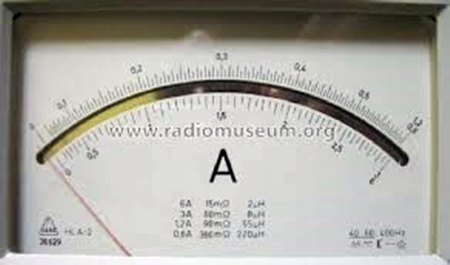## Classification or Types of Ammeter

Depending on the constructing principle, there are many types of ammeter we get, they are mainly –

1. Permanent Magnet Moving Coil(PMMC) ammeter.
2. Moving Iron (MI) Ammeter.
3. Electrodynamometer type Ammeter.
4. Rectifier type Ammeter.

Depending on this types of measurement we do, we have-

1. DC Ammeter.
2. AC Ammeter.

DC Ammeter are mainly PMMC instruments, MI can measure both AC and DC currents, also Electrodynamometer type thermal instrument can measure DC and AC, induction meters are not generally used for ammeter construction due to their higher cost, inaccuracy in measurement.

## Description of Different Types of Ammeters

### PMMC Ammeter

Principle PMMC Ammeter:
When current carrying conductor placed in a magnetic field, a mechanical force acts on the conductor, if it is attached to a moving system, with the coil movement, the pointer moves over the scale.
Explanation: As the name suggests it has permanent magnets which are employed in this kind of measuring instruments. It is particularly suited for DC measurement because here deflection is proportional to the current and hence if current direction is reversed, deflection of the pointer will also be reversed so it is used only for DC measurement. This type of instrument is called D Arnsonval type instrument. It has major advantage of having linear scale, low power consumption, high accuracy. Major disadvantage of being measured only DC quantity, higher cost etc.
Deflecting torque,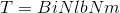Where,
B = Flux density in Wb/m².
i = Current flowing through the coil in Amp.
l = Length of the coil in m.
b = Breadth of the coil in m.
N = No of turns in the coil.
Extension of Range in a PMMC Ammeter:
Now it looks quite extraordinary that we can extend the range of measurement in this type of instrument. Many of us will think that we must buy a new ammeter to measure higher amount of current and also many of us may think we have to change the constructional feature so that we can measure higher currents, but there is nothing like that, we just have to connect a shunt resistance in parallel and the range of that instrument can be extended, this is a simple solution provided by the instrument.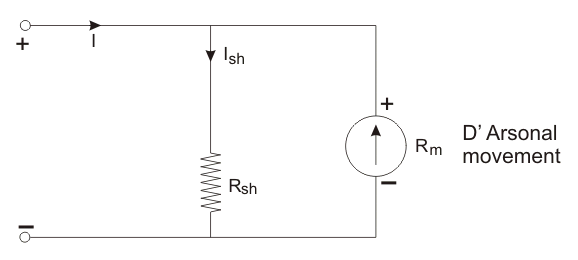In the figure I = total current flowing in the circuit in Amp.
Ish is the current through the shunt resistor in Amp.
Rm is the ammeter resistance in Ohm.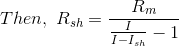### MI Ammeter

It is a moving iron instrument, used for both AC and DC, It can be used for both because the deflection θ proportional square of the current so whatever is the direction of current, it shows directional deflection, further they are classified in two more ways-

1. Attraction type.
2. Repulsion type.

Its torque equation is: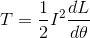Where,
I is the total current flowing in the circuit in Amp.
L is the self inductance of the coil in Henry.
θ is the deflection in Radian.

1. Attraction Type MI Instrument Principle:
When an unmagnetised soft iron is placed in the magnetic field, it is attracted towards the coil, if a moving system attached and current is passed through a coil, it creates a magnetic field which attracts iron piece and creates deflecting torque as a result of which pointer moves over the scale.
2. Repulsion Type MI Instrument Principle:
When two iron pieces are magnetized with same polarity by passing a current than repulsion between them occurs and that repulsion produces a deflecting torque due to which the pointer moves.
The advantages of MI instruments are they can measure both AC and DC, cheap, low friction errors, robustness etc. It is mainly used in AC measurement because in DC measurement error will be more due to hysteresis.

### Electrodynamometer Type Ammeter

This can be used to measure both i.e. AC and DC currents. Now we see that we have PMMC and MI instrument for the measurement of AC and DC currents, a question may arise – “why do we need Electrodynamometer Ammeter? If we can measure current accurately by other instrument also?”. The answer is Electrodynamometer instruments have the same calibration for both AC and DC i.e. if it is calibrated with DC, then also without calibrating we can measure AC.

Principle Electrodynamometer Type Ammeter:
There we have two coils, namely fixed and moving coils. If a current is passed through two coils it will stay in the zero position due to the development of equal and opposite torque. If somehow, the direction of one torque is reversed as the current in the coil reverses, an unidirectional torque is produced.
For ammeter, the connection is a series one and φ = 0
Where, φ is the phase angle.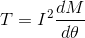Where,
I is the amount of current flowing in the circuit in Amp.
M = Mutual inductance of the coil.
They have no hysteresis error, used for both AC and DC measurement, the main disadvantages are they have low torque/weight ratio, high friction loss, expensive than other measuring instruments etc.

### Rectifier Ammeter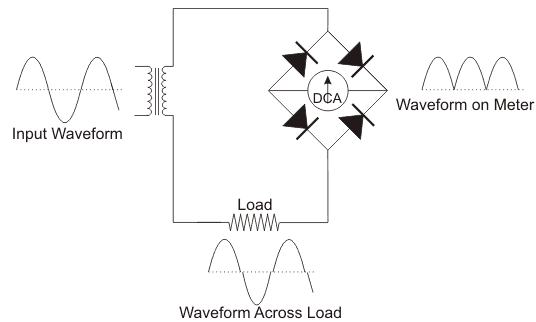Principle of Rectifier Ammeter:
They are used for AC measurement which is connected to secondary of a current transformer, the secondary current is much less than primary and connected with a bridge rectifier to a moving coil ammeter.

1. It can be used in high frequency also.
2. Uniform scale for most of the ranges.

Disadvantages being error due to temperature decrease in sensitivity in AC operation.

Want To Learn Faster? 🎓
Get electrical articles delivered to your inbox every week.
No credit card required—it’s 100% free.Electrical4U is dedicated to the teaching and sharing of all things related to electrical and electronics engineering.

### 2 thoughts on “Ammeter Working Principle and Types of Ammeter”

1.Very good explanation .

•